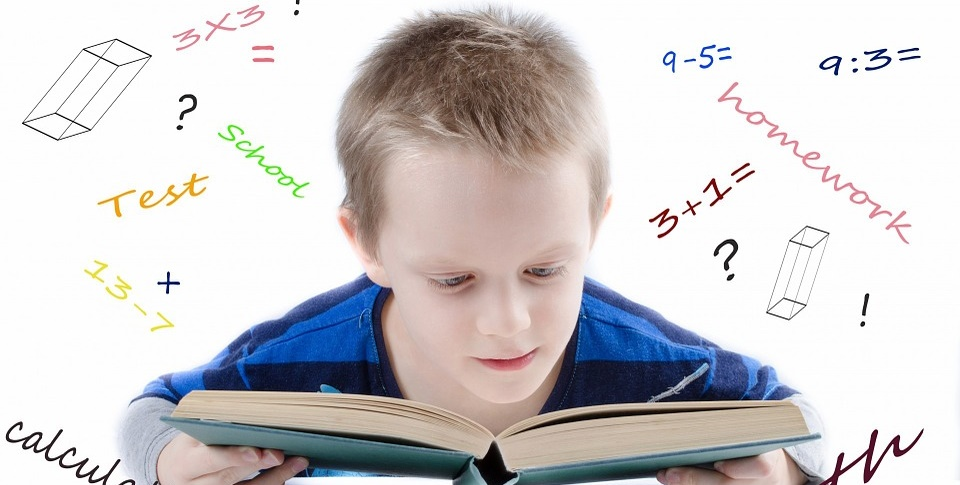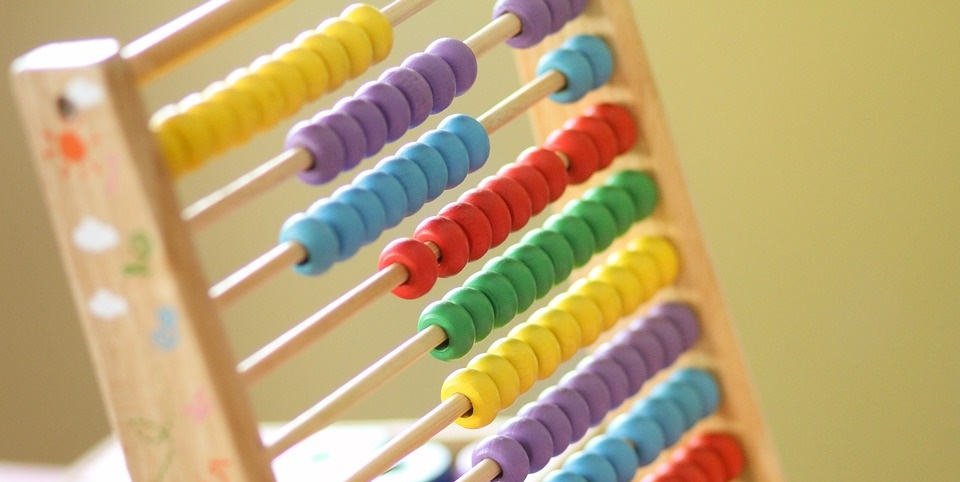# Maths

## Add, Subtract, Multiply, Divide & Fractions

• Third Grade Math: Add two numbers together, then check yourself by subtraction. Divide two numbers and check yourself by multiplication. In third grade, you learn about equations and their relationships.
• Math Baseball: Funbrain lets you take a swing at math problems. If you get them right, you get hits. If not, then you’re out!
• Practicing Math Facts: Practice makes perfect! You can time yourself if you want while getting the hang of addition, subtraction, multiplication and division.
• Subtraction Bowling: Solve the problem, throw the bowling ball until all pins are down.
• Fractions: Do you know how to add and subtract, multiply and divide fractions? What is a fraction? What fractions equal each other?
• Multiplication Problems: Use red, yellow, green and blue Lego bricks to help you see how to solve multiplication equations.
• Skip Counting: Count the oars by groups to complete this quiz.
• Fractions and Decimals: Ways to remember how to double or half fractions and mixed numbers.
• Fraction Equivalents: Find a new way to name the fraction.
• Decimal Jeopardy: Compare and practice number patterns with
• Subtraction Tunnel Blaster: Shoot the wall with the right subtraction problem answer or create a hole in your ship.## Algebra & Geometry

• Absurd Math: Solve this very cool math problem and you just might save the planet.
• Real World Math Videos: See, just don’t read about, coordinate systems, points, lines, shapes and angles.
• What’s a Tangram? Learn about geometric shapes by constructing Chinese tangrams.
• Space Figures: Geometry for kids by kids, this particular one is about 3D space figures.
• Integers and Algebra: Sixth-grade level lessons about integers, equations and expressions, patterns, functions and coordinates.
• Patterns, Shapes and Fractions: Use patterns to find out what fractions of shapes will be a whole shape.
• 3D Geometric Drawing: An introduction to the prism, pyramid, cylinder and cone combinations that can be used to make beautiful 3D drawings.
• Geometry Formula References: Formulas for area and circumference, volume and surface area. Includes some advanced formulas if you’re ready to learn them!
• Basic Algebra Formulas and More: Keep this “cheat sheet” handy for homework when you have to work basic algebra and exponent problems. If you are working in advanced math, there are formulas here for you as well.
• Pascal’s Pumpkins: Can you find the sequences in Pascal’s pumpkin?
• Simplifying Algebraic Expressions: This tutorial takes you step-by-step to learn about combining like terms in order to simply your algebra.
• Rewriting Algebra: Write algebraic expressions for the word expression.

## Counting Money

• Counting Money: Add the values of the coins and put the amount in the blank to see if you are correct.
• Money Words: Every letter of the alphabet has a coin value. Find the value of each word.
• Slide Coins: Let the coins roll down the slide into the cup until you reach the value of money at the top.
• CA\$H OUT: You are the store’s cashier. It’s up to you to make change before the time runs out.
• Counting Change: Match the money amount with its word amount.
• Money Addition Count: Click on the money in the cashier’s drawer to solve the story problem.
• Coin Memory Game: Match the coins in these six memory games.
• Counting Money With Kristen: Kristen wants to buy a teddy bear, books and some balloons. Can you help her count her change?
• Visit Fedville: Create your own character and password to visit Fedville and your character’s home. See how your character deals with money.
• The Piggy Bank: Learn and then practice counting coins with some neat tricks from first grade teacher, Ms. Ross.
• Planet Orange: Follow Cedric and Amy in their quest to learn about saving and spending money on Planet Orange.
• Buy It With Little Farmer: Count out the money to buy food from the farmer’s market.## Math Puzzles

• Stick Triangle: Can you remove two sticks to make 10 triangles?
• Tetris: Fit the shapes inside the rectangle to win the game.
• Sudoku: Play these two Sudoku math puzzles: one challenge and one just plain fun.
• Favorite Math Puzzles: Lots of fun puzzles—some easy, some will confuse you and your friends!
• Sixteen Pennies: Can you leave an equal number of pennies in each row just by removing six of them?
• Button Beach Challenge: Solve the matrix puzzle. Pass three levels to win the challenge.
• Sliders: Move the numbers around the slider until they are in order to complete the puzzle.
• Birthday Guessing Game: Can you solve this logic problem and find whose birthday belongs to whom?
• Scrambled Equations: Can you make 42 out of 2, 3, 4 and 9?
• Puzzles and Problems: Fifth graders—have fun solving these puzzles and word problems.
• Tangram Puzzles: Solve the people puzzle.
• Math Vocabulary Puzzle: Have fun solving this crossword puzzle that will help you learn your math terms.

## Statistics & Probability

• The Canadian Census Word Search: Learn about the Canadian census. A census is a count of people that happens once every 10 years in the United States. People are counted in Canada’s census once every five years, although we both live in North America.
• Create a Graph: Graphs communicate visually. If you have to graph how many people eat lunch at school during one week, you can learn to graph that statistic as a bar, line, pie, dot or area graph.
• Spotting Statistical Lies: How to know if the statistics you are seeing are real or poorly represented. You should know what mean, median and mode definitions are.
• Data to Graphics: See how numbers relate to pictures and images that represent them.
• The Mean Machine: Click on the motorbike to play. Each bike is numbered 1 to 9. Find the mean, median and range of the three bikes.
• The Birthday Problem: Find the probability that two people in your class (or in another group of people) have the same birthday by using this probability calculator.
• Data and Probability Words: Read the definition and choose the math word that matches.
• Cyberchase Coin Toss: Would you get more heads or more tails if you tossed a coin 100 times? Let’s check it out and see what happens.
• Everybody Counts: Matching game for the U.S. Census for Kids. Match all the cards and reveal a cool fact about people.
• Animal Island Data: Tally the island animals so you can gather data on them later.
• Understanding Statistics: These will help you visualize and understand about histograms, the area, mean average and variance of data. For you older elementary students getting into the big guy stuff!
• Statistics a la Mode: Can you find the ice cream statistic mode?